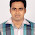## Pages

### Prime Number

```/* Prime Number using while loop */

#include<stdio.h>
int main()
{
int a,i,f;
printf("Enter a number: ");
scanf("%d",&a);
f=0;
i=2;
while(i <= a/2)
{
if(a%i == 0)
{
f=1;
break;
}
i++;
}
if(f==0)
printf("Prime Number")
else
printf("Not Prime Number");
return 0;
}

-------------------------------------------------------

/* Prime number using for loop */

#include<stdio.h>
int main()
{
int a,i,f;
printf("Enter a number: ");
scanf("%d",&a);
f=0;
for(i=2;i <= a/2;i++)
{
if(a%i == 0)
{
f=1;
break;
}
}
if(f==0)
printf("Prime Number")
else
printf("Not Prime Number");
return 0;
}
```

1.This comment has been removed by the author.

2.#include
#include
int isPrime(long num)
{
int i,num_sqrt=sqrt(num)+1;
if(num<2) return 0;
if(num==2 || num==3) return 1;
if(num%2==0 || num%3==0) return 0;
for(i=6;i<=num_sqrt;i+=6)
if(num%(i-1)==0 || num%(i+1)==0) return 0;
return 1;
}

int main()
{
int num;
printf("Enter a number :");
scanf("%d",&num);
if(isPrime(num))
printf("Prime number");
else
printf("Not prime number");
return 0;
}

3.there is an error in the program

expected ; before else

#include
int main()
{
int a,i,f;
printf("Enter a number: ");
scanf("%d",&a);
f=0;
for(i=2;i <= a/2;i++)
{
if(a%i == 0)
{
f=1;
break;
}
}
if(f==0)
printf("Prime Number");
else
printf("Not Prime Number");
return 0;
}

4.This comment has been removed by the author.

5.#include
#include

void main()

{ //start of main ()
int i,j,n,count;
clrscr();
printf("WAP to find out all prime numbers between range.\n");
printf("-----------------------------------------------");
printf("\nEnter the staring range :");
scanf("%d",&i);
printf("\nEnter the ending range :");
scanf("%d",&n);
//-------------------------------------
if(i==n && i>2 && n>2) //when 2 inputs were same then
{
printf("\nPrime numbers are listed below:");
int c=0;
for(int j=2;j<=n/2;j++)
{
if(n%j==0)
{
c++;
break;
}
}
if(c>0)
{
printf("\nThere are no Prime numbers between your range.");
}
else
printf("\n%d",n);

} //end of if
//-------------------------------------
else if(i<=n && i>=0 && n>1) //if condition start for check i1) //if condition for print the values
{
printf("%d \t",i);
} //end the if condition

} //end of loop that shows it reach the ending range
} //end of if for checking i<n

//----------------------------------------
else //print wrong input from user
{
printf("\n*Your ending value should be greater than 1.\n**Ending value should be equal or greater than Starting value.\n***This program does not support negative values.");
}

getch();
} //end of main()

1.hey author, when i am publishing my whole code then some codes does not uploads, some codes are mismatched at uploading,